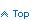Loop through an ASP.NET Datagrid and retrieve cell values

You can programmatically retrieve ASP.NET DataGrid cell values from your VB.NET or C# codebehind by looping through the DataGrid as shown in the following code snippets. The codebehind in these samples was written in VB.NET.

Assume we have a DataGrid with a simple Last Name BoundColumn that contains plain text. We also have a TemplateColumnTemplateColumn that contains a TextBox so users can enter a percentage amount. Notice that in the percent column the String.Format method is used to format the value. Lastly, we have a column labeled Select which is a TemplateColumn that displays a CheckBox.

```<asp:datagrid id="MyGrid" runat="server" ....>
...
<Columns>
<ItemStyle HorizontalAlign="Center" ...></ItemStyle>
</asp:BoundColumn>
<ITEMSTYLE HORIZONTALALIGN="Center" ...></ITEMSTYLE>
<ITEMTEMPLATE>
<asp:TextBox runat="server" Width="60px" MaxLength="6"
Text='<%# String.Format("{0:f2}",
DataBinder.Eval(Container, "DataItem.PCT")) %>'
ID="txtPercent">
</asp:TextBox>
</ITEMTEMPLATE>
</ASP:TEMPLATECOLUMN>
<ITEMSTYLE HORIZONTALALIGN="Center"...></ITEMSTYLE>
<ITEMTEMPLATE>
<asp:CheckBox runat="server" ID="chkSelect" Checked='False' />
</ITEMTEMPLATE>
</ASP:TEMPLATECOLUMN>
</Columns>
</asp:datagrid>
```

Now, in our server code we want to loop through the DataGrid and sum up the percentages where the CheckBoxes are checked. First, we define some variables. We need to define a DataGridItem variable to hold a row of the ASP.NET DataGrid.

```  Dim intRow As Integer = 0
Dim strName As String = ""
Dim strPercent As String = ""
Dim dblTotal As Double = 0
Dim dblPercent As Double = 0
'
' Number of rows in the DataGrid
'
Dim intRows As Integer = MyGrid.Items.Count - 1
Dim blnChecked As Boolean = False
'
' DataGrid rows are really DataGridItems.
'
Dim GridItem As DataGridItem
```

Now we can loop through the DataGrid rows. To retrieve the value of the Last Name column we can just reference the cell directly. Columns or cells in a DataGridItem are zero based. However, to get the TextBox and CheckBox values we need to use the FindControl method to locate the control in the DataGridItem using its ID. Then we need to cast it to the correct type. This is done using the DirectCast function although the slower but safer CType method could have been used.

```  For intRow = 0 To intRows
'
' Get the grid row.
'
item = MyGrid.Items(intRow)
'
' Get the Last Name
'
strName = item.Cells(0).Text().Trim()
'
' Find the controls in the DataGrid row and get their values.
'
strPercent = DirectCast(item.FindControl("txtPercent"), TextBox).Text().Trim()
blnChecked = DirectCast(item.FindControl("chkSelect"), CheckBox).Checked

If blnChecked Then
If IsNumeric(strPercent) Then
dblPercent = Convert.ToSingle(strPercent)
dblTotal += dblPercent
End If
End If
Next
```

Suppose you wanted to add a button to the web page that calculates the total of the percentages just like above but you want this done in the browser on the client side. You can do this with some JavaScript.

Add an HTML button to the web page. Use it's OnClick event to fire the Calculate JavaScript function.

```  <INPUT id="cmdSumPct" title="Calculate the total percent"
onclick="Calculate();" type="button" value="Calculate Total" name="cmdSumPct">
```

Now, you need to look at the actual HTML generated by .NET by running your code, bringing up your web page and viewing its source. What you need to determine is what the actual names (IDs) are for the Form, DataGrid, TextBox and CheckBox controls. Using the above names, they should be as shown below. Note that JavaScript is case sensitive.

Here is the JavaScript function to calculate the total percent. This needs to go in your web pages <HEAD>: tag.

```  <SCRIPT language="JavaScript">
function Calculate()
{
var idRoot    = 'MyGrid__ctl';
var idtxtTail = '_txtPercent';
var idchkTail = '_chkSelect';
//
// The first grid row will have an index of 2.
//
var count   = 2;
var total   = 0.0;
var txtctrl = null;
var chkctrl = null;
//
// Get the first TextBox.
//
txtctrl = document.getElementById(idRoot + count + idtxtTail);

while (txtctrl != null)
{
//
// Get the CheckBox.
//
chkctrl = document.getElementById(idRoot + count + idchkTail);
if (chkctrl.checked)
{
total += parseFloat(txtctrl.value);
}
//
// Get the next TextBox.
//
count++
txtctrl = document.getElementById(idRoot + count + idtxtTail);
}
document.Form1.txtTotal.value = total;
}
</SCRIPT>
```

You can play around with this and add error handling to the server code as well as the client-side JavaScript. You can also call the Calculate function when the CheckBox is checked or unchecked.